# 3. Baseline models & k-Nearest Neighbours¶

## 3.1. Lecture Learning Objectives¶

• Use DummyClassifier and DummyRegressor as baselines for machine learning problems.

• Explain the notion of similarity-based algorithms .

• Broadly describe how KNNs use distances.

• Discuss the effect of using a small/large value of the hyperparameter $$K$$ when using the KNN algorithm

• Describe the problem of the curse of dimensionality.

## 3.2. Five Minute Recap/ Lightning Questions¶

• What are the 4 types of data/splits that we discussed last class?

• What is the “Golden Rule of Machine Learning”?

• What do we use to split our data?

• What is overfitting and underfitting?

### 3.2.1. Some lingering questions¶

• Are decision trees the most basic model?

• What other models can we build?

## 3.3. Baseline Models¶

We saw in the last 2 lectures how to build decision tree models which are based on rules (if-else statements), but how can we be sure that these models are doing a good job besides just accuracy?

Back in high school in chemistry or biology, we’ve all likely seen and heard of the “control group” where we have an experimental group, does not receive any experimental treatment. This control group increases the reliability of the results, often through a comparison between control measurements and the other measurements.

Our baseline model is something like a control group in the sense that it provides a way to sanity-check your machine learning model. We make baseline models not to use for prediction purposes, but as a reference point when we are building other more sophisticated models.

So what is a baseline model then?

• Baseline: A simple machine learning algorithm based on simple rules of thumb. For example,

• most frequent baseline: always predicts the most frequent label in the training set.

### 3.3.1. Dummy Classifier¶

We are going to build a most frequent baseline model which always predicts the most frequently labelled in the training set.

import pandas as pd

voting_df

lon lat vote
1 -80.162475 25.692104 blue
2 -80.214360 25.944083 blue
3 -80.094133 26.234314 blue
4 -80.248086 26.291902 blue
5 -81.789963 26.348035 blue
... ... ... ...
396 -97.460476 48.225094 red
397 -96.551116 48.591592 blue
398 -166.519855 53.887114 red
399 -163.733617 67.665859 red
400 -145.423115 68.077395 red

400 rows × 3 columns

# feature table
X = voting_df.drop(columns='vote')

# the target variable
y = voting_df[['vote']]

from sklearn.model_selection import cross_validate, train_test_split

X_train, X_test, y_train, y_test = train_test_split(
X, y, test_size=0.2, random_state=123)


We build our model, in the same way as we built a decision tree model but this time using DummyClassifier.

Since we are using a “most frequent” baseline model, we specify the argument strategy as "most_frequent"

There are other options that you you read about in the sklearn documentation but you just need to know most_frequent for now.

from sklearn.dummy import DummyClassifier

dummy_clf = DummyClassifier(strategy="most_frequent")


In the last lecture, we stated that it’s at this point that we would usually perform cross-validation to evaluate the model performance.

With Dummy Classifiers, we won’t need to because we are not hyperparameter tuning. We are using this just to get a base training score.

dummy_clf.fit(X_train, y_train)
dummy_clf.score(X_train, y_train)

0.578125


If we see what our model predicts on the feature table for our training split X_train, our model will predict the most occurring class from our training data.

y_train.value_counts()

vote
blue    185
red     135
dtype: int64

dummy_clf.predict(X_train)

array(['blue', 'blue', 'blue', 'blue', 'blue', 'blue', 'blue', 'blue',
'blue', 'blue', 'blue', 'blue', 'blue', 'blue', 'blue', 'blue',
'blue', 'blue', 'blue', 'blue', 'blue', 'blue', 'blue', 'blue',
'blue', 'blue', 'blue', 'blue', 'blue', 'blue', 'blue', 'blue',
'blue', 'blue', 'blue', 'blue', 'blue', 'blue', 'blue', 'blue',
'blue', 'blue', 'blue', 'blue', 'blue', 'blue', 'blue', 'blue',
'blue', 'blue', 'blue', 'blue', 'blue', 'blue', 'blue', 'blue',
'blue', 'blue', 'blue', 'blue', 'blue', 'blue', 'blue', 'blue',
'blue', 'blue', 'blue', 'blue', 'blue', 'blue', 'blue', 'blue',
'blue', 'blue', 'blue', 'blue', 'blue', 'blue', 'blue', 'blue',
'blue', 'blue', 'blue', 'blue', 'blue', 'blue', 'blue', 'blue',
'blue', 'blue', 'blue', 'blue', 'blue', 'blue', 'blue', 'blue',
'blue', 'blue', 'blue', 'blue', 'blue', 'blue', 'blue', 'blue',
'blue', 'blue', 'blue', 'blue', 'blue', 'blue', 'blue', 'blue',
'blue', 'blue', 'blue', 'blue', 'blue', 'blue', 'blue', 'blue',
'blue', 'blue', 'blue', 'blue', 'blue', 'blue', 'blue', 'blue',
'blue', 'blue', 'blue', 'blue', 'blue', 'blue', 'blue', 'blue',
'blue', 'blue', 'blue', 'blue', 'blue', 'blue', 'blue', 'blue',
'blue', 'blue', 'blue', 'blue', 'blue', 'blue', 'blue', 'blue',
'blue', 'blue', 'blue', 'blue', 'blue', 'blue', 'blue', 'blue',
'blue', 'blue', 'blue', 'blue', 'blue', 'blue', 'blue', 'blue',
'blue', 'blue', 'blue', 'blue', 'blue', 'blue', 'blue', 'blue',
'blue', 'blue', 'blue', 'blue', 'blue', 'blue', 'blue', 'blue',
'blue', 'blue', 'blue', 'blue', 'blue', 'blue', 'blue', 'blue',
'blue', 'blue', 'blue', 'blue', 'blue', 'blue', 'blue', 'blue',
'blue', 'blue', 'blue', 'blue', 'blue', 'blue', 'blue', 'blue',
'blue', 'blue', 'blue', 'blue', 'blue', 'blue', 'blue', 'blue',
'blue', 'blue', 'blue', 'blue', 'blue', 'blue', 'blue', 'blue',
'blue', 'blue', 'blue', 'blue', 'blue', 'blue', 'blue', 'blue',
'blue', 'blue', 'blue', 'blue', 'blue', 'blue', 'blue', 'blue',
'blue', 'blue', 'blue', 'blue', 'blue', 'blue', 'blue', 'blue',
'blue', 'blue', 'blue', 'blue', 'blue', 'blue', 'blue', 'blue',
'blue', 'blue', 'blue', 'blue', 'blue', 'blue', 'blue', 'blue',
'blue', 'blue', 'blue', 'blue', 'blue', 'blue', 'blue', 'blue',
'blue', 'blue', 'blue', 'blue', 'blue', 'blue', 'blue', 'blue',
'blue', 'blue', 'blue', 'blue', 'blue', 'blue', 'blue', 'blue',
'blue', 'blue', 'blue', 'blue', 'blue', 'blue', 'blue', 'blue',
'blue', 'blue', 'blue', 'blue', 'blue', 'blue', 'blue', 'blue',
'blue', 'blue', 'blue', 'blue', 'blue', 'blue', 'blue', 'blue',
'blue', 'blue', 'blue', 'blue', 'blue', 'blue', 'blue', 'blue'],
dtype='<U4')


We can also now take the test score.

dummy_clf.score(X_test, y_test)

0.6125


Here is a good example of when we occasionally have test scores better than the training scores.

In this case, it’s higher because our test split has a higher proportion of observations that are of class blue and so more of them will be predicted correctly.

Now if we build a decision tree, we can compare it to the baseline model score.

Here we use crossvalidation to score the model although we are not tuning any hyperparameters. It is general a good idea to get into the habit of doing this for all models except baseline/dummy models, because the split can influence the model score (this is true to a much lesser extent for baseline models)

from sklearn.tree import DecisionTreeClassifier

dt_clf = DecisionTreeClassifier()

scores = cross_validate(dt_clf, X_train, y_train, cv=10, return_train_score=True)
pd.DataFrame(scores).mean()[-2:]

test_score     0.884375
train_score    1.000000
dtype: float64

dt_clf.fit(X_train, y_train)
dt_clf.score(X_test, y_test)

0.8875


We can see that our decision tree is doing better than a model build on this simple “most frequently” occurring model. This makes us trust our model a little more and we know that it has identified some structure in the data that is more complicated than simply predicting the most common label. This is especially important if we have an unbalanced training data set with much more of one label than of another, but there are also more sophisticated strategies to handle that situation, which we will discuss later.

### 3.3.2. Dummy Regressor¶

For a Dummy regressor, the same principles can be applied but by using different strategies.

“mean”, “median”, “quantile”, “constant”

The one we are going to become most familiar with is:

Average (mean) target value: always predicts the mean of the training set.

house_df = pd.read_csv("data/kc_house_data.csv")
house_df = house_df.drop(columns=["id", "date"])
house_df

price bedrooms bathrooms sqft_living sqft_lot floors waterfront view condition grade sqft_above sqft_basement yr_built yr_renovated zipcode lat long sqft_living15 sqft_lot15
0 221900.0 3 1.00 1180 5650 1.0 0 0 3 7 1180 0 1955 0 98178 47.5112 -122.257 1340 5650
1 538000.0 3 2.25 2570 7242 2.0 0 0 3 7 2170 400 1951 1991 98125 47.7210 -122.319 1690 7639
2 180000.0 2 1.00 770 10000 1.0 0 0 3 6 770 0 1933 0 98028 47.7379 -122.233 2720 8062
3 604000.0 4 3.00 1960 5000 1.0 0 0 5 7 1050 910 1965 0 98136 47.5208 -122.393 1360 5000
4 510000.0 3 2.00 1680 8080 1.0 0 0 3 8 1680 0 1987 0 98074 47.6168 -122.045 1800 7503
... ... ... ... ... ... ... ... ... ... ... ... ... ... ... ... ... ... ... ...
21608 360000.0 3 2.50 1530 1131 3.0 0 0 3 8 1530 0 2009 0 98103 47.6993 -122.346 1530 1509
21609 400000.0 4 2.50 2310 5813 2.0 0 0 3 8 2310 0 2014 0 98146 47.5107 -122.362 1830 7200
21610 402101.0 2 0.75 1020 1350 2.0 0 0 3 7 1020 0 2009 0 98144 47.5944 -122.299 1020 2007
21611 400000.0 3 2.50 1600 2388 2.0 0 0 3 8 1600 0 2004 0 98027 47.5345 -122.069 1410 1287
21612 325000.0 2 0.75 1020 1076 2.0 0 0 3 7 1020 0 2008 0 98144 47.5941 -122.299 1020 1357

21613 rows × 19 columns

Let get our X and y objects and split our data.

X = house_df.drop(columns=["price"])
y = house_df["price"]


We still need to make sure we split our data with baseline models.

X_train, X_test, y_train, y_test = train_test_split(
X, y, test_size=0.2, random_state=123)


we need to import DummyRegressor and construct our model.

We specify strategy="mean" however this is the default value so technically we don’t need to specify this.

We train our model and again, it’s not needed to cross-validate for this type of algorithm.

from sklearn.dummy import DummyRegressor

dummy_reg = DummyRegressor(strategy="mean")
dummy_reg.fit(X_train,y_train)

DummyRegressor()


If we predict on our training data, we see it’s making the same prediction for each observation.

dummy_reg.predict(X_train)

array([539306.46784268, 539306.46784268, 539306.46784268, ...,
539306.46784268, 539306.46784268, 539306.46784268])


if we compare the mean value of the target, we see that our model is simply predicting the average of the training data which is exactly what we expect.

y_train.mean()

539306.4678426837


How well does it do?

dummy_reg.score(X_train, y_train)

0.0


We get an $$R^2$$ value of 0.0.

When a model has an $$R^2$$=0 that means that the model is doing no better than a model that using the mean which is exactly the case here.

Looking at the test score we see that our model get’s a slightly negative value, which means that it is doing a little worse than constantly predicting the mean of the test data.

dummy_reg.score(X_test, y_test)

-0.00010814646015933072


## 3.4. Let’s Practice¶

1. Below we have the output of y_train.value_counts()

Position
Forward     13
Defense      7
Goalie       2
dtype: int64


In this scenario, what would a DummyClassifier(strategy='most_frequent') model predict on the following observation:

   No.  Age  Height  Weight  Experience     Salary
1   83   34     191     210          11  3200000.0


2. When using a regression model, which of the following is not a possible return value from .score(X,y) ?

a) 0.0
b) 1.0
c) -0.1
d) 1.5

3. Below are the values for y that were used to train DummyRegressor(strategy='mean'):

Grade
0     75
1     80
2     90
3     95
4     85
dtype: int64


What value will the model predict for every example?

## 3.5. Similarity/analogy-based models¶

Let’s switch topics from baseline models and talked about the concept of similarity based models.

Suppose you are given the following training images with the corresponding actor’s name as the label. Then we are given an unseen test images of the same actors but from different angles and are asked to label a given test example.

An intuitive way to classify the test example is by finding the most “similar” example from the training images and use that label for the test example.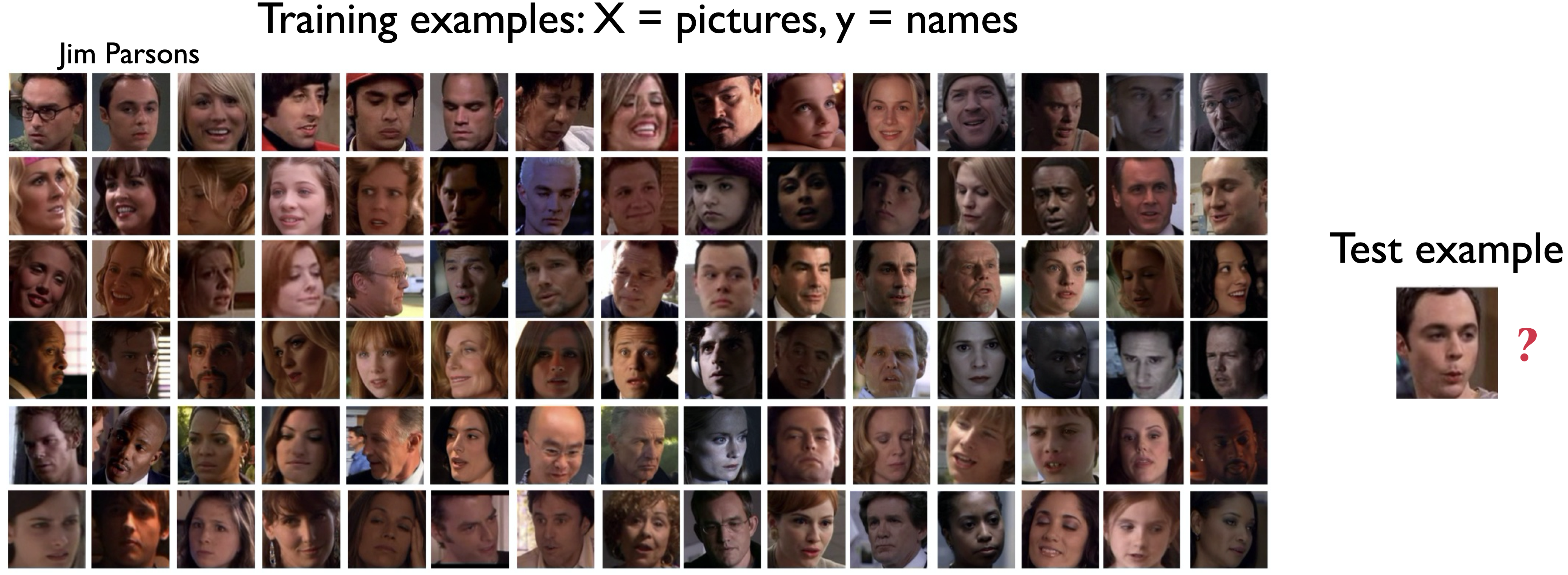### 3.5.1. Example:¶

Suppose we are given many images and their labels.

X = set of pictures

y = names associated with those pictures.

Then we are given a new unseen test example, a picture in this particular case.We want to find out the label for this new test picture.

Naturally, we would try and find the most similar picture in our training set and using the label of the most similar picture as the label of this new test example.

That’s the basic idea behind similarity/analogy-based algorithms.

This is different from Decision trees, where we tried to learn a set of rules/conditions to label observations correctly.

### 3.5.2. Similarity/Analogy-based models in real life¶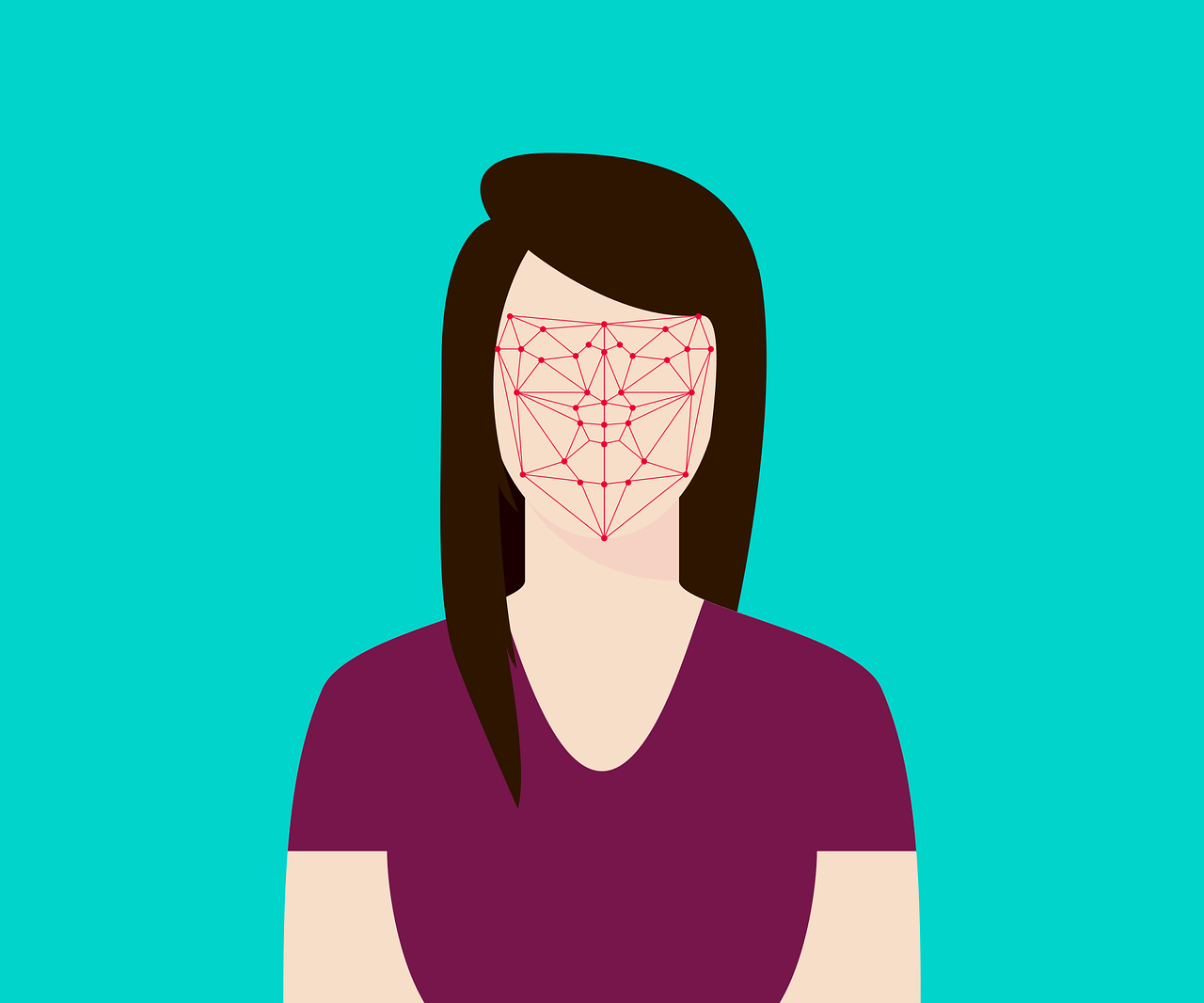• Recommendation systems## 3.6. Terminology¶

In analogy-based algorithms, our goal is to come up with a way to find similarities between examples. “similarity” is a bit ambiguous so we need some terminology.

• data: think of observations (rows) as points in a high dimensional space.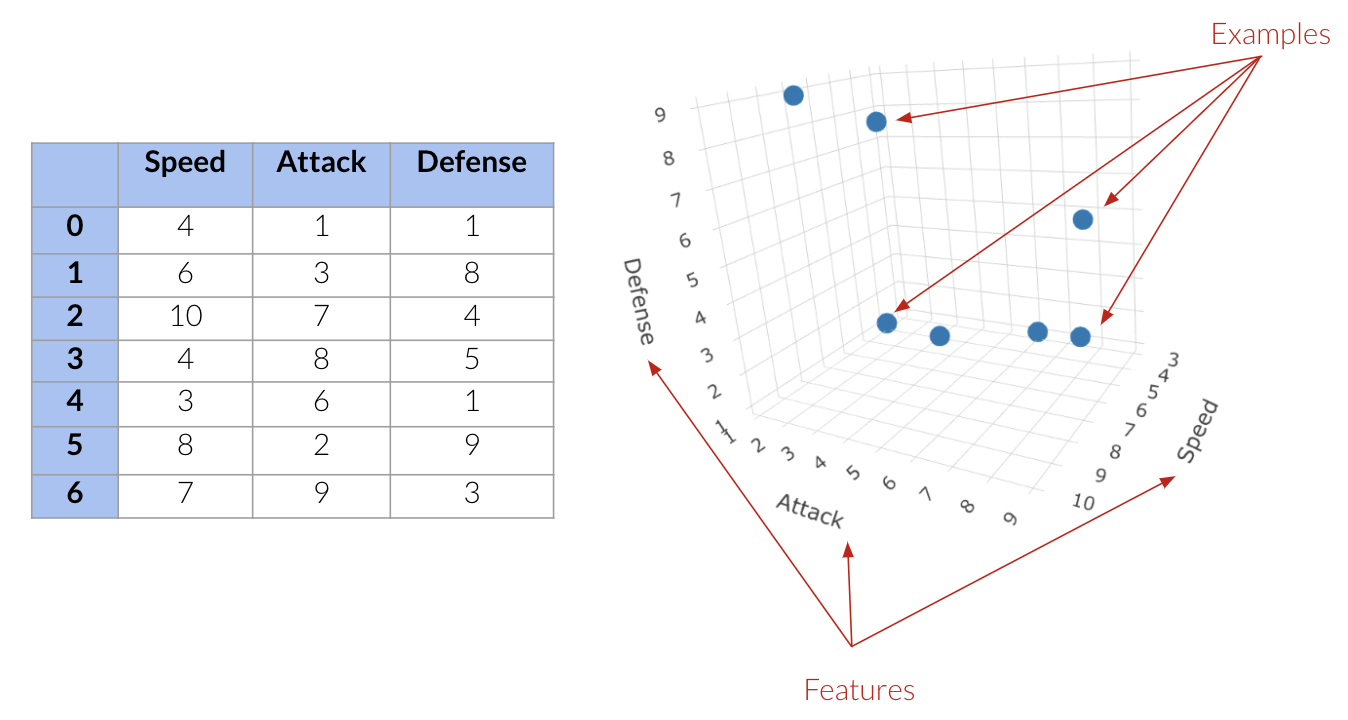Above we have:

• Three features; speed attack and defense.

• 7 points in this three-dimensional space.

Let’s go back to our Canada/USA cities dataset.

cities_df = pd.read_csv("data/canada_usa_cities.csv")
cities_train_df, cities_test_df = train_test_split(cities_df, test_size=0.2, random_state=123)
cities_train_df

longitude latitude country
... ... ... ...
17 -76.3305 44.1255 USA
66 -121.4944 38.5816 USA

167 rows × 3 columns

We have 2 features, so 2 dimensions (longitude and latitude) and 167 points. Visualizing this in 2 dimensions gives us the following:

import altair as alt

cities_viz = alt.Chart(cities_train_df).mark_circle(size=20, opacity=0.6).encode(
alt.X('longitude:Q', scale=alt.Scale(domain=[-140, -40])),
alt.Y('latitude:Q', scale=alt.Scale(domain=[20, 60])),
range=['red', 'blue']))
)
cities_viz


What about the housing training dataset we saw?

house_df = pd.read_csv("data/kc_house_data.csv")
house_df = house_df.drop(columns=["id", "date"])

X = house_df.drop(columns=["price"])
y = house_df["price"]

house_X_train, house_X_test, house_y_train, house_y_test = train_test_split(
X, y, test_size=0.2, random_state=123)

house_X_train

bedrooms bathrooms sqft_living sqft_lot floors waterfront view condition grade sqft_above sqft_basement yr_built yr_renovated zipcode lat long sqft_living15 sqft_lot15
14305 3 2.75 2170 2738 1.5 0 0 4 9 1550 620 1930 0 98109 47.6389 -122.349 1170 1062
19844 4 2.75 3550 9400 2.0 0 0 3 9 3550 0 2014 0 98059 47.4827 -122.131 3550 9421
2686 3 3.25 1210 1173 2.0 0 0 3 8 1000 210 2002 0 98133 47.7114 -122.356 1650 1493
11926 3 2.50 2370 7875 2.0 0 0 3 9 2370 0 2003 0 98065 47.5427 -121.863 2660 7752
13873 5 1.75 2250 8970 1.0 0 0 4 7 1500 750 1966 0 98034 47.7217 -122.188 1940 8710
... ... ... ... ... ... ... ... ... ... ... ... ... ... ... ... ... ... ...
15377 4 2.50 3440 14554 2.0 1 4 3 8 2170 1270 2012 0 98155 47.7364 -122.286 3170 11810
21602 3 1.75 1500 11968 1.0 0 0 3 6 1500 0 2014 0 98010 47.3095 -122.002 1320 11303
17730 2 1.00 1640 5200 1.0 0 0 4 7 1040 600 1937 0 98199 47.6426 -122.403 1780 5040
15725 3 3.00 1560 1466 3.0 0 0 3 8 1560 0 2006 0 98103 47.6604 -122.352 1530 2975
19966 4 2.50 2300 3825 2.0 0 0 3 7 2300 0 2012 0 98042 47.3594 -122.082 2110 3825

17290 rows × 18 columns

Notice a problem?!

We can only visualize data when the dimensions <= 3.

BUT, in ML, we usually deal with high-dimensional problems where examples are hard to visualize. High dimensional = 100s or 1000s of dimensions (roughly speaking, it depends on the specific problem at hands and the density of the data).

### 3.6.1. Feature Vectors¶

Feature vector: a vector composed of feature values associated with an example.

An example feature vector from the cities dataset:

cities_train_df.drop(columns=["country"]).iloc.to_numpy()

array([-76.4813,  44.2307])


An example feature vector from the housing dataset:

house_X_train.iloc.to_numpy()

array([ 3.00000e+00,  2.75000e+00,  2.17000e+03,  2.73800e+03,
1.50000e+00,  0.00000e+00,  0.00000e+00,  4.00000e+00,
9.00000e+00,  1.55000e+03,  6.20000e+02,  1.93000e+03,
0.00000e+00,  9.81090e+04,  4.76389e+01, -1.22349e+02,
1.17000e+03,  1.06200e+03])


## 3.7. Distance¶

We have our feature vectors, one for each observation, but how we calculate the similarity between these feature vectors?

One way to calculate the similarity between two points in high-dimensional space is by calculating the distance between them.

So, if the distance is higher, that means that the points are less similar and when the distance is smaller, that means that the points are more similar.

### 3.7.1. Euclidean distance¶

There are different ways to calculate distance but we are going to focus on Euclidean distance.

Euclidean distance: Euclidean distance is a measure of the true straight line distance between two points in Euclidean space. (source)

The Euclidean distance between vectors

$$$u = <u_1, u_2, \dots, u_n>$$$ and

$$$v = <v_1, v_2, \dots, v_n>$$$ is defined as:

$$distance(u, v) = \sqrt{\sum_{i =1}^{n} (u_i - v_i)^2}$$

Because that equation can look a bit intimidating, let’s use it in an example, particularly our Canadian/US cities data.

#### 3.7.1.1. Calculating Euclidean distance “by hand”¶

cities_train_df

longitude latitude country
... ... ... ...
17 -76.3305 44.1255 USA
66 -121.4944 38.5816 USA

167 rows × 3 columns

Let’s take 2 points (two feature vectors) from the cities dataset.

two_cities = cities_df.sample(2, random_state=42).drop(columns=["country"])
two_cities

longitude latitude
30 -66.9843 44.8607
171 -80.2632 43.1408

The two sampled points are shown as black circles below.

Our goal is to find how similar these two points are.

cities_viz + alt.Chart(two_cities).mark_circle(size=130, color='black').encode(
alt.X('longitude'),
alt.Y('latitude')
)


First, we subtract these two cities. We are subtracting the city at index 0 from the city at index 1.

two_cities.iloc - two_cities.iloc

longitude   -13.2789
latitude     -1.7199
dtype: float64


Next, we square the differences.

(two_cities.iloc - two_cities.iloc)**2

longitude    176.329185
latitude       2.958056
dtype: float64


Then we sum up the squared differences.

((two_cities.iloc - two_cities.iloc)**2).sum()

179.28724121999983


And then take the square root of the value.

# To the power of 0.5 is the same as square root
((two_cities.iloc - two_cities.iloc)**2).sum()**0.5

13.389818565611703


We end with a value of 13.3898 which is the distance between the two cities in feature space.

#### 3.7.1.2. Calculating Euclidean distance with sklearn¶

That’s more work than we really have time for and since sklearn knows we are very busy people, they have a function that does this for us.

from sklearn.metrics.pairwise import euclidean_distances

euclidean_distances(two_cities)

array([[ 0.        , 13.38981857],
[13.38981857,  0.        ]])


When we call this function on our two cities data, it outputs this matrix with four values.

• Our first value is the distance between city 0 and itself.

• Our second value is the distance between city 0 and city1.

• Our third value is the distance between city 1and city 0.

• Our fourth value is the distance between city 1 and itself.

As we can see, the distances are symmetric. If we calculate the distance between city 0 and city1, it’s going to have the same value as if we calculated the distance between city 1 and city 0.

This isn’t always the case if we use a different metric to calculate distances.

## 3.8. Finding the Nearest Neighbour¶

Now that we know how to calculate the distance between two points, we can use this metric as a definition for what “most similar” means in our cities data. In this case, we would say that it is the cities that have the shortest distance between them. These are often called the “Nearest Neighbors”.

Let’s find the closest cities to City 0 from our cities_train_df dataframe.

Using euclidean_distances on the entire dataset will calculate the distances from all the cities to all other cities in our dataframe.

dists = euclidean_distances(cities_train_df[["latitude", "longitude"]])
dists

array([[ 0.        ,  4.92866046, 10.47586257, ..., 45.36619339,
3.13968038,  9.58476504],
[ 4.92866046,  0.        , 15.36399019, ..., 40.48484175,
1.80868018, 14.45684087],
[10.47586257, 15.36399019,  0.        , ..., 55.83947468,
13.60621684,  0.94361393],
...,
[45.36619339, 40.48484175, 55.83947468, ...,  0.        ,
42.23325838, 54.93872568],
[ 3.13968038,  1.80868018, 13.60621684, ..., 42.23325838,
0.        , 12.70774745],
[ 9.58476504, 14.45684087,  0.94361393, ..., 54.93872568,
12.70774745,  0.        ]])


This is going to be of shape 167 by 167 as this was the number of examples in our training portion.

Each row here gives us the distance of that particular city to all other cities in the training data.

dists.shape

(167, 167)

pd.DataFrame(dists)

0 1 2 3 4 5 6 7 8 9 ... 157 158 159 160 161 162 163 164 165 166
0 0.000000 4.928660 10.475863 3.402295 9.046000 44.329135 31.525721 3.212817 8.167347 26.529757 ... 0.180478 39.498997 46.632397 9.154090 46.632397 0.183869 1.921478 45.366193 3.139680 9.584765
1 4.928660 0.000000 15.363990 8.326614 13.965788 39.839439 26.601406 2.099390 4.686255 21.937558 ... 5.097647 34.577431 42.060108 14.063632 42.060108 5.049876 6.830969 40.484842 1.808680 14.456841
2 10.475863 15.363990 0.000000 7.195350 2.653738 54.549042 41.853597 13.661189 18.304496 36.104309 ... 10.299402 49.928916 56.925712 1.397385 56.925712 10.337038 8.674609 55.839475 13.606217 0.943614
3 3.402295 8.326614 7.195350 0.000000 5.643921 47.391337 34.926888 6.501805 11.133646 29.822103 ... 3.239187 42.880277 49.747776 5.833864 49.747776 3.295760 1.503112 48.707466 6.527458 6.347179
4 9.046000 13.965788 2.653738 5.643921 0.000000 52.532333 40.567164 12.088727 16.408137 35.345303 ... 8.883025 48.487730 54.961957 1.674158 54.961957 8.938714 7.134836 54.253225 12.162108 2.483804
... ... ... ... ... ... ... ... ... ... ... ... ... ... ... ... ... ... ... ... ... ...
162 0.183869 5.049876 10.337038 3.295760 8.938714 44.493704 31.639843 3.370041 8.341824 26.596728 ... 0.090157 39.625372 46.793616 9.021147 46.793616 0.000000 1.833858 45.502887 3.270795 9.442309
163 1.921478 6.830969 8.674609 1.503112 7.134836 45.960223 33.432373 5.001225 9.708835 28.425488 ... 1.768291 41.377557 48.300994 7.323990 48.300994 1.833858 0.000000 47.206600 5.028487 7.810194
164 45.366193 40.484842 55.839475 48.707466 54.253225 12.149226 15.249886 42.205693 38.013270 24.755170 ... 45.541763 6.789646 10.695838 54.518494 10.695838 45.502887 47.206600 0.000000 42.233258 54.938726
165 3.139680 1.808680 13.606217 6.527458 12.162108 41.375773 28.405702 0.756038 5.558903 23.675006 ... 3.312655 36.359838 43.637580 12.291750 43.637580 3.270795 5.028487 42.233258 0.000000 12.707747
166 9.584765 14.456841 0.943614 6.347179 2.483804 53.731902 40.924593 12.780661 17.476788 35.161267 ... 9.407212 49.012123 56.094462 0.842200 56.094462 9.442309 7.810194 54.938726 12.707747 0.000000

167 rows × 167 columns

The distance of each city to itself is going to be zero.

If we don’t replace the 0’s on the diagonal with infinity, each city’s most similar city is going to be itself which is not useful.

import numpy as np

np.fill_diagonal(dists, np.inf)
pd.DataFrame(dists)

0 1 2 3 4 5 6 7 8 9 ... 157 158 159 160 161 162 163 164 165 166
0 inf 4.928660 10.475863 3.402295 9.046000 44.329135 31.525721 3.212817 8.167347 26.529757 ... 0.180478 39.498997 46.632397 9.154090 46.632397 0.183869 1.921478 45.366193 3.139680 9.584765
1 4.928660 inf 15.363990 8.326614 13.965788 39.839439 26.601406 2.099390 4.686255 21.937558 ... 5.097647 34.577431 42.060108 14.063632 42.060108 5.049876 6.830969 40.484842 1.808680 14.456841
2 10.475863 15.363990 inf 7.195350 2.653738 54.549042 41.853597 13.661189 18.304496 36.104309 ... 10.299402 49.928916 56.925712 1.397385 56.925712 10.337038 8.674609 55.839475 13.606217 0.943614
3 3.402295 8.326614 7.195350 inf 5.643921 47.391337 34.926888 6.501805 11.133646 29.822103 ... 3.239187 42.880277 49.747776 5.833864 49.747776 3.295760 1.503112 48.707466 6.527458 6.347179
4 9.046000 13.965788 2.653738 5.643921 inf 52.532333 40.567164 12.088727 16.408137 35.345303 ... 8.883025 48.487730 54.961957 1.674158 54.961957 8.938714 7.134836 54.253225 12.162108 2.483804
... ... ... ... ... ... ... ... ... ... ... ... ... ... ... ... ... ... ... ... ... ...
162 0.183869 5.049876 10.337038 3.295760 8.938714 44.493704 31.639843 3.370041 8.341824 26.596728 ... 0.090157 39.625372 46.793616 9.021147 46.793616 inf 1.833858 45.502887 3.270795 9.442309
163 1.921478 6.830969 8.674609 1.503112 7.134836 45.960223 33.432373 5.001225 9.708835 28.425488 ... 1.768291 41.377557 48.300994 7.323990 48.300994 1.833858 inf 47.206600 5.028487 7.810194
164 45.366193 40.484842 55.839475 48.707466 54.253225 12.149226 15.249886 42.205693 38.013270 24.755170 ... 45.541763 6.789646 10.695838 54.518494 10.695838 45.502887 47.206600 inf 42.233258 54.938726
165 3.139680 1.808680 13.606217 6.527458 12.162108 41.375773 28.405702 0.756038 5.558903 23.675006 ... 3.312655 36.359838 43.637580 12.291750 43.637580 3.270795 5.028487 42.233258 inf 12.707747
166 9.584765 14.456841 0.943614 6.347179 2.483804 53.731902 40.924593 12.780661 17.476788 35.161267 ... 9.407212 49.012123 56.094462 0.842200 56.094462 9.442309 7.810194 54.938726 12.707747 inf

167 rows × 167 columns

Now let’s look at the distance between city 0 and some other cities.

We can look at city 0 with its respective longitude and latitude values.

cities_train_df.iloc[]

longitude latitude country

And the distances from city 0 to the other cities in the training dataset.

dists

array([        inf,  4.92866046, 10.47586257,  3.40229467,  9.04600003,
44.32913545, 31.52572108,  3.21281701,  8.16734667, 26.52975665,
5.36451793, 17.47354208,  9.25235538, 46.65300827, 12.91711643,
8.73732272, 21.41984759, 46.10101237, 11.38925694,  8.45258153,
3.29603996, 12.90216965, 25.43541008,  2.57167487, 35.80191816,
54.77569987,  2.77064993,  4.74762925, 21.11653758, 46.84711517,
18.63306299, 42.68410294, 21.26505054, 46.58691036, 23.62784332,
4.45224202,  4.47636916, 36.52381145,  9.25295786,  6.83445279,
3.32357247,  8.16734667, 31.19662805,  7.79242035,  1.42424148,
23.99849627, 38.17792497,  4.94149717, 21.5518279 ,  9.51787313,
38.19275017, 37.97055885,  2.97880445,  9.51483002, 37.1901251 ,
54.83532899,  1.28350735, 10.0044146 ,  3.28029451, 45.92971431,
11.01430039,  3.19225293, 46.38943202,  2.96693525,  9.04600003,
35.80001702,  2.76505999,  3.07231406, 36.96774058, 38.19559327,
3.10919556,  7.39488827,  2.95943349, 11.50351059,  3.93684711,
42.2793155 ,  9.14918312,  6.83445279, 46.91610836, 43.38757936,
3.5288519 , 46.38746533,  3.93581626,  2.96373905, 21.27058643,
18.66494501, 26.50025275,  6.82764827,  4.7396424 ,  9.9874779 ,
6.83549241, 13.13067545, 46.50627115, 26.49966844, 28.81491391,
45.97271218, 46.90321547,  5.73649067, 59.62262041,  1.09761148,
6.08870392,  5.73454545, 46.21089504,  9.03328701,  7.92447701,
23.80155222, 47.07123507,  8.73468637,  2.74816746,  3.07662193,
3.22855068,  3.63284771, 19.68277227,  2.82337311,  3.43691007,
7.93860597, 46.85396601, 16.3288186 , 46.23882897, 43.94670281,
28.85319232,  2.91163454,  2.88081958, 16.80079704, 23.7934676 ,
3.9286773 ,  2.86603875,  3.32357247,  1.07865087,  8.4376414 ,
6.82764827,  3.0717763 ,  4.45272866,  9.11228649,  4.08772282,
2.86603875, 34.6881178 ,  1.8400926 ,  3.03326165, 23.32224009,
46.10101237, 46.83865522, 22.78600867, 28.85530775, 18.81002995,
46.69549579, 13.44345687, 17.34527477,  6.75905672,  5.89141512,
3.13784784,  3.47624672, 42.98365051, 43.00228242, 10.65641958,
3.80625889,  1.42924216,  0.18047839, 39.49899714, 46.63239678,
9.15409025, 46.63239678,  0.18386865,  1.92147809, 45.36619339,
3.13968038,  9.58476504])


Remember that our goal is to find the closest example to city 0.

We can find the closest city to city 0 by finding the city with the minimum distance.

np.argmin(dists)

157


The closest city in the training dataset is the city with index 157.

This corresponds to index 96 from the original dataset before shuffling.

cities_train_df.iloc[]

longitude latitude country

If we look at the longitude and latitude values for the city at index 157 (labelled 96), they look pretty close to those of city 0.

cities_train_df.iloc[]

longitude latitude country
dists

0.18047839205805613


So, in this case, the closest city to city 0 is city 157 and the Euclidean distance between the two cities is 0.184.

### 3.8.1. Nearest city to a query point¶

We can also find the distances to a new “test” or “query” city:

query_point = [[-80, 25]]

dists = euclidean_distances(cities_train_df[["longitude", "latitude"]], query_point)
dists[:10]  # Only show the first few observations

array([[19.54996348],
[18.02706204],
[24.60912622],
[21.39718237],
[25.24111312],
[47.81750619],
[28.49499735],
[19.39177482],
[21.95316686],
[19.01698738]])


We can find the city closest to the query point (-80, 25) using:

np.argmin(dists)

147

dists[np.argmin(dists)]

array([3.83839229])


So the city at index 147 is the closest point to (-80, 25) with the Euclidean distance between the two equal to 3.838

Instead of doing this manually we can use Sklearn’s NearestNeighbors function to get the closest example and the distance between the query point and the closest example.

from sklearn.neighbors import NearestNeighbors

nn = NearestNeighbors(n_neighbors=1)
nn.fit(cities_train_df[['longitude', 'latitude']]);
nn.kneighbors([[-80, 25]])

/home/joel/miniconda3/envs/bait/lib/python3.9/site-packages/sklearn/base.py:450: UserWarning: X does not have valid feature names, but NearestNeighbors was fitted with feature names
warnings.warn(

(array([[3.83839229]]), array([]))


We can also use kneighbors to find the 5 nearest cities in the training split to one of the cities in the test split.

cities_test_X = cities_test_df[['longitude', 'latitude']]

nn = NearestNeighbors(n_neighbors=5)
nn.fit(cities_train_df[['longitude', 'latitude']]);
nn.kneighbors(cities_test_X.iloc[])

(array([[0.03461517, 0.90722048, 0.90722048, 0.90970871, 0.90970871]]),
array([[100,  77,  39,  87, 130]]))


On the last line, we need to be careful and make sure that we pass in either a 2D numpy array or a dataframe as our input. This is why we use 2 sets of square brackets with our city above.

## 3.9. Let’s Practice¶

       seeds   shape  sweetness   water-content      weight    fruit_veg
0      1        0        35          84               100        fruit
1      0        0        23          75               120        fruit
2      1        1        15          90              1360         veg
3      1        1         7          96               600         veg
4      0        0        37          80                 5        fruit
5      0        0        45          78                40        fruit
6      1        0        27          83               450         veg
7      1        1        18          73                 5         veg
8      1        1        32          80                76         veg
9      0        0        40          83                65        fruit


1. Giving the table above and that we are trying to predict if each example is either a fruit or a vegetable, what would be the dimension of feature vectors in this problem?

2. Which of the following would be the feature vector for example 0.

a) array([1,  0, 1, 1, 0, 0, 1, 1, 1, 0])
b) array([fruit,  fruit, veg, veg, fruit, fruit, veg, veg, veg, fruit])
c) array([1, 0, 35, 84, 100])
d) array([1, 0, 35, 84, 100,  fruit])

3. Given the following 2 feature vectors, what is the Euclidean distance between the following two feature vectors?

u = np.array([5, 0, 22, -11])
v = np.array([-1, 0, 19, -9])


True or False

4. Analogy-based models find examples from the test set that are most similar to the test example we are predicting.
5. Feature vectors can only be of length 3 since we cannot visualize past that.
6. Euclidean distance will always have a positive value.
7. When finding the nearest neighbour in a dataset using kneighbors() from the sklearn library, we must fit the data first.

## 3.10. $$k$$ -Nearest Neighbours ($$k$$-NNs) Classifier¶

Now that we have learned how to find similar examples, can we use this idea in a predictive model?

• Yes! The k Nearest Neighbors (kNN) algorithm

• This is a fairly simple algorithm that is best understood by example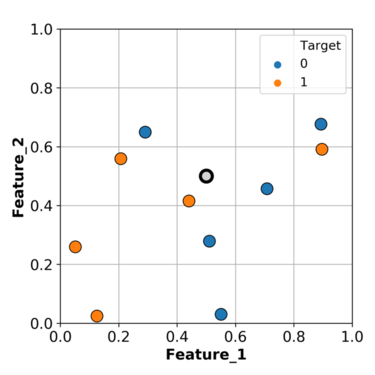We have two features in our toy example; feature 1 and feature 2.

We have two targets; 0 represented with blue points and 1 represented with orange points.

We want to predict the point in gray.

Based on what we have been doing so far, we can find the closest example ($$k$$=1) to this gray point and use its class as the class for our grey point.

In this particular case, we will predict orange as the class for our query point.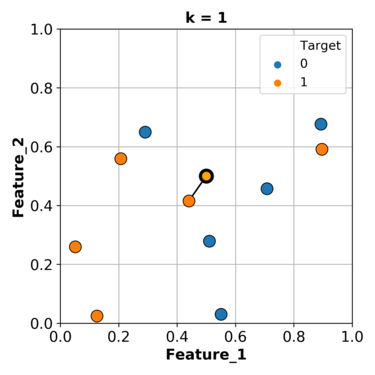What if we consider more than one nearest example and let them vote on the target of the query example.

Let’s consider the nearest 3 neighbours and let them vote.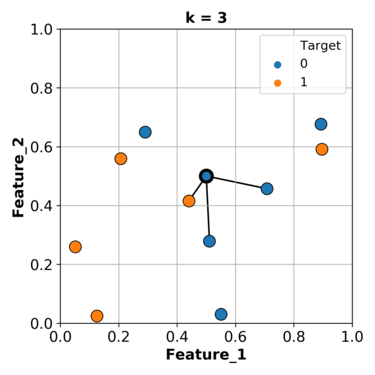Let’s try this with a smaller set of our data and sklearn.

small_train_df = cities_train_df.sample(30, random_state=1223)
small_X_train = small_train_df.drop(columns=["country"])
small_y_train = small_train_df["country"]

one_city = cities_test_df.sample(1, random_state=33)
one_city

longitude latitude country
chart_knn = alt.Chart(small_train_df).mark_circle().encode(
alt.X('longitude', scale=alt.Scale(domain=[-140, -40])),
alt.Y('latitude', scale=alt.Scale(domain=[20, 60])),

one_city_point = alt.Chart(one_city).mark_point(
shape='triangle-up', size=400, fill='darkgreen', opacity=1).encode(
alt.X('longitude'),
alt.Y('latitude')
)

chart_knn +  one_city_point


We want to find the class for this green triangle city.

from sklearn.neighbors import KNeighborsClassifier

neigh_clf = KNeighborsClassifier(n_neighbors=1)
neigh_clf.fit(small_X_train, small_y_train)
neigh_clf.predict(one_city.drop(columns=["country"]))

array(['Canada'], dtype=object)


We can set n_neighbors equal to 1 to classify this triangle based on one neighbouring point.

Our prediction here is Canada since the closest point to the green triangle is a city with the class “Canada”.

Now, what if we consider the nearest 3 neighbours?

neigh_clf = KNeighborsClassifier(n_neighbors=3)
neigh_clf.fit(small_X_train, small_y_train)
neigh_clf.predict(one_city.drop(columns=["country"]))

array(['USA'], dtype=object)


When we change our model to consider the nearest 3 neighbours, our prediction changes!

It now predicts “USA” since the majority of the 3 nearest points are “USA” cities.

Let’s use our entire training dataset and calculate our training and validation scores

cities_X_train = cities_train_df.drop(columns=['country'])
cities_y_train = cities_train_df['country']
cities_X_test = cities_test_df.drop(columns=['country'])
cities_y_test = cities_test_df['country']

kn1_model = KNeighborsClassifier(n_neighbors=1)
scores = cross_validate(kn1_model, cities_X_train, cities_y_train, cv=10, return_train_score=True)

scores_df = pd.DataFrame(scores)
scores_df

fit_time score_time test_score train_score
0 0.002475 0.002093 0.823529 1.0
1 0.001399 0.001869 0.705882 1.0
2 0.001503 0.001856 0.647059 1.0
3 0.001508 0.001797 0.941176 1.0
4 0.001377 0.001621 0.823529 1.0
5 0.001268 0.001399 0.588235 1.0
6 0.001389 0.001456 0.647059 1.0
7 0.001302 0.001423 0.812500 1.0
8 0.001266 0.001498 0.937500 1.0
9 0.001331 0.001545 0.750000 1.0
scores_df.mean()

fit_time       0.001482
score_time     0.001656
test_score     0.767647
train_score    1.000000
dtype: float64


## 3.11. Choosing K¶

Ok, so we saw our validation and training scores for n_neighbors =1. What happens when we change that?

kn90_model = KNeighborsClassifier(n_neighbors=90)

scores_df = pd.DataFrame(cross_validate(kn90_model, cities_X_train, cities_y_train, cv=10, return_train_score=True))
scores_df

fit_time score_time test_score train_score
0 0.001924 0.002541 0.705882 0.620000
1 0.001278 0.002043 0.588235 0.620000
2 0.001830 0.002455 0.588235 0.633333
3 0.001358 0.001940 0.588235 0.606667
4 0.001391 0.001709 0.588235 0.620000
5 0.001365 0.001626 0.588235 0.633333
6 0.001363 0.001619 0.647059 0.700000
7 0.001348 0.001807 0.625000 0.629139
8 0.001831 0.002362 0.687500 0.622517
9 0.002112 0.002652 0.625000 0.629139
scores_df.mean()

fit_time       0.001580
score_time     0.002075
test_score     0.623162
train_score    0.631413
dtype: float64


Comparing this with the results of n_neighbors=1 we see that we went from overfitting to underfitting.

Let’s look at the decision boundaries now.

from plot_classifier import plot_classifier
import matplotlib.pyplot as plt

plt.figure(figsize=(16, 8))
plt.subplot(1, 2, 1)
kn1_model.fit(cities_X_train, cities_y_train);
plt.title("n_neighbors = 1")
plt.ylabel("latitude")
plt.xlabel("longitude")
plot_classifier(cities_X_train, cities_y_train, kn1_model, ax=plt.gca(), ticks=True)

plt.subplot(1, 2, 2)
plt.title("n_neighbors = 90")
kn90_model.fit(cities_X_train, cities_y_train);
plt.ylabel("latitude")
plt.xlabel("longitude")
plot_classifier(cities_X_train, cities_y_train, kn90_model, ax=plt.gca(), ticks=True)

/home/joel/miniconda3/envs/bait/lib/python3.9/site-packages/sklearn/base.py:450: UserWarning: X does not have valid feature names, but KNeighborsClassifier was fitted with feature names
warnings.warn(
/home/joel/miniconda3/envs/bait/lib/python3.9/site-packages/sklearn/base.py:450: UserWarning: X does not have valid feature names, but KNeighborsClassifier was fitted with feature names
warnings.warn(

<AxesSubplot:title={'center':'n_neighbors = 90'}, xlabel='longitude', ylabel='latitude'>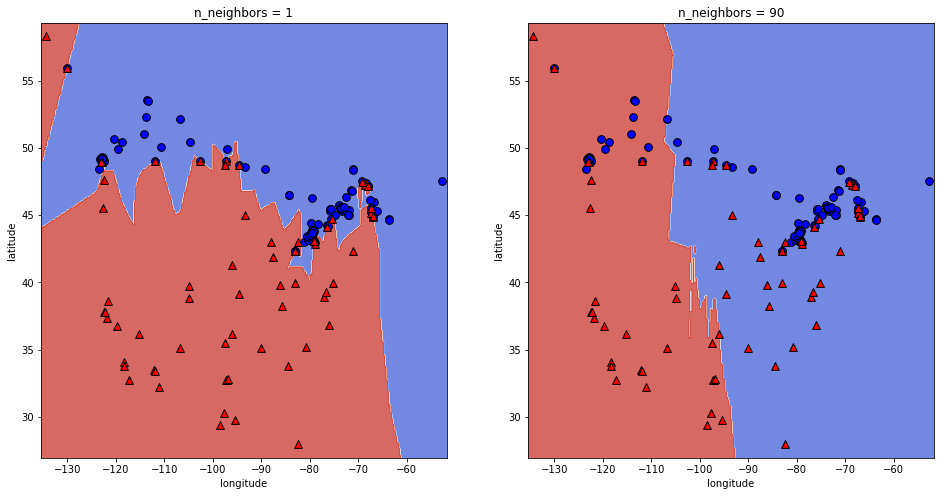If we plot these two models with $$k=1$$ on the left and $$k=90$$ on the right.

The left plot shows a much more complex model where it is much more specific and attempts to get every example correct.

The plot on right is plotting a simpler model and we can see more training examples are being predicted incorrectly.

### 3.11.1. How to choose $$K$$ (n_neighbors)?¶

So we saw the model was overfitting with $$k$$=1 and when $$k$$=90, the model was underfitting.

So, the question is how do we pick $$k$$?

• Since $$k$$ is a hyperparameter (n_neighbors in sklearn), we can use hyperparameter optimization to choose $$k$$.

Here we are looping over different values of $$k$$ and performing cross-validation on each one.

results_dict = {"n_neighbors": list(), "mean_train_score": list(), "mean_cv_score": list()}

for k in range(1, 50, 5):
knn = KNeighborsClassifier(n_neighbors=k)
scores = cross_validate(knn, cities_X_train, cities_y_train, cv=10, return_train_score=True)
results_dict["n_neighbors"].append(k)
results_dict["mean_cv_score"].append(np.mean(scores["test_score"]))
results_dict["mean_train_score"].append(np.mean(scores["train_score"]))

results_df = pd.DataFrame(results_dict)
results_df

n_neighbors mean_train_score mean_cv_score
0 1 1.000000 0.767647
1 6 0.841660 0.785662
2 11 0.823051 0.811029
3 16 0.807726 0.793382
4 21 0.777810 0.756985
5 26 0.765854 0.739338
6 31 0.758525 0.738971
7 36 0.738583 0.720221
8 41 0.725912 0.726471
9 46 0.733916 0.713971
plotting_source = results_df.melt(
id_vars='n_neighbors',
value_vars=['mean_train_score', 'mean_cv_score'],
var_name='score_type',
value_name='accuracy'
)

K_plot = alt.Chart(plotting_source, width=500, height=300).mark_line().encode(
alt.X('n_neighbors:Q'),
alt.Y('accuracy:Q', scale=alt.Scale(domain=[.67, 1.00])),
alt.Color('score_type:N')
).properties(title="Accuracies of n_neighbors for KNeighborsClassifier")

K_plot


Looking at this graph with k on the x-axis and accuracy on the y-axis, we can see there is a sweet spot where the gap between the validation and training scores is the lowest and cross-validation score is the highest. Here it’s when n_neighbors is 11.

How do I know it’s 11? Here’s how!

results_df.sort_values("mean_cv_score", ascending=False)

n_neighbors mean_train_score mean_cv_score
2 11 0.823051 0.811029
3 16 0.807726 0.793382
1 6 0.841660 0.785662
0 1 1.000000 0.767647
4 21 0.777810 0.756985
5 26 0.765854 0.739338
6 31 0.758525 0.738971
8 41 0.725912 0.726471
7 36 0.738583 0.720221
9 46 0.733916 0.713971
knn = KNeighborsClassifier(n_neighbors=11)
knn.fit(cities_X_train, cities_y_train);
print("Test accuracy:", round(knn.score(cities_X_test, cities_y_test), 3))

Test accuracy: 0.857


This testing accuracy even higher than the validation mean accuracy we had earlier.

## 3.12. Curse of Dimensionality¶

$$k$$ -NN usually works well when the number of dimensions is small.

In the previous module, we discussed one of the most important problems in machine learning which was overfitting. The second most important problem in machine learning is the curse of dimensionality. This refers to that datasets with many many features generally have a sparser distribution of observations within this feature space, since there are so many possible unique combinations of features. This makes it both harder to detect the same general patterns between observations in the data and easier to accidentally mistake “noise” (meaningless correlations) for general patterns.

We will talk more later about what can be done to alleviate the curse of dimensionality, but for now it is enough to know that many supervised ML algorithms, including k-NN, can perform poorly when there are too high dimensionality in the data. With enough irrelevant features, the accidental similarity between features wipes out any meaningful similarity and $$k$$-NN is no better than random guessing.

## 3.13. Let’s Practice¶

Consider this toy dataset:

$\begin{split} X = \begin{bmatrix}5 & 2\\4 & 3\\ 2 & 2\\ 10 & 10\\ 9 & -1\\ 9& 9\end{bmatrix}, \quad y = \begin{bmatrix}A\\A\\B\\B\\B\\C\end{bmatrix}.\end{split}$

What would you predict for $$x=\begin{bmatrix} 0 & 0\end{bmatrix}$$:

1. If $$k=1$$?
2. If $$k=3$$?

True or False

3. The classification of the closest neighbour to the test example always contributes the most to the prediction.
4. The n_neighbors hyperparameter must be less than the number of examples in the training set.
5. Similar to decision trees, $$k$$-NNs find a small set of good features.
6. With $$k$$-NN, setting the hyperparameter $$k$$ to larger values typically increases training score.
7. $$k$$-NN may perform poorly in high-dimensional space (say, d > 100)
8. Based on the graph below, what value of n_neighbors would you choose to train your model on?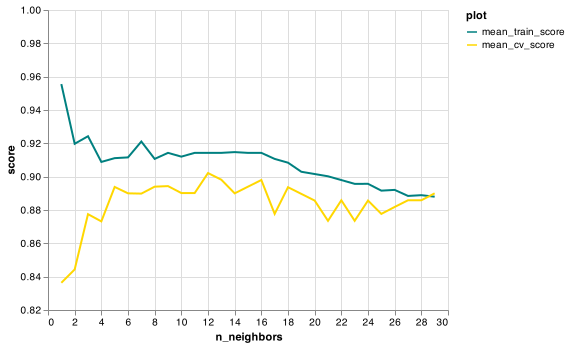## 3.14. What We’ve Learned Today¶

• The concept of baseline models.

• How to initiate a Dummy Classifier and Regressor.

• How to measure Euclidean distance.

• How the $$k$$NN algorithm works for classification.

• How changing $$k$$ (n_neighbors) affects a model.

• What the curse of dimensionality is.